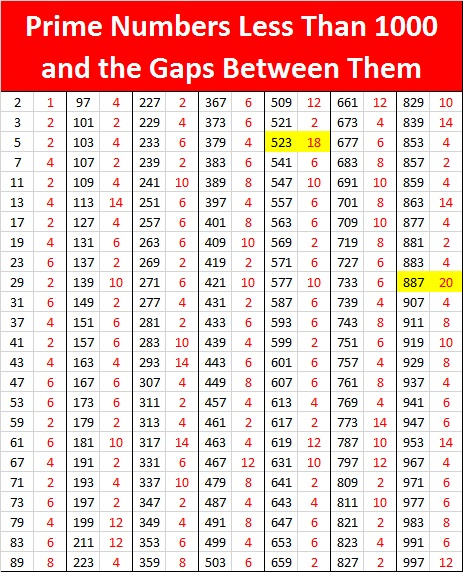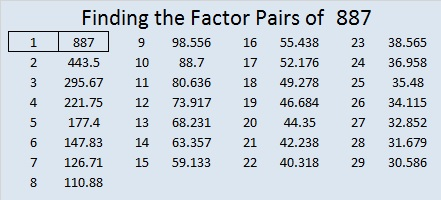887 is the Last Prime Number for a While!

The last prime number before 887 was 883.

The next prime number won’t be until 907. Wow!907 – 887 = 20. That’s the largest gap between prime numbers so far!

887 is also a palindrome in a few other bases:

• 31313 BASE 4, because 3(4⁴) + 1(4³) + 3(4²) + 1(4) + 3(1) = 887
• 737 BASE 11, because 7(11²) + 3(11) + 7(1) = 887
• 313 BASE 17, because 3(17²) + 1(17) + 3(1) = 887

Those patterns of 3’s and 1’s for two different bases surprised me!

• 887 is a prime number.
• Prime factorization: 887 is prime.
• The exponent of prime number 887 is 1. Adding 1 to that exponent we get (1 + 1) = 2. Therefore 887 has exactly 2 factors.
• Factors of 887: 1, 887
• Factor pairs: 887 = 1 × 887
• 887 has no square factors that allow its square root to be simplified. √887 ≈ 29.782545

How do we know that 887 is a prime number? If 887 were not a prime number, then it would be divisible by at least one prime number less than or equal to √887 ≈ 29.8. Since 887 cannot be divided evenly by 2, 3, 5, 7, 11, 13, 17, 19, 23, or 29, we know that 887 is a prime number.